# 单样本学习（One shot learning）和孪生网络（Siamese Network）简介

## 背景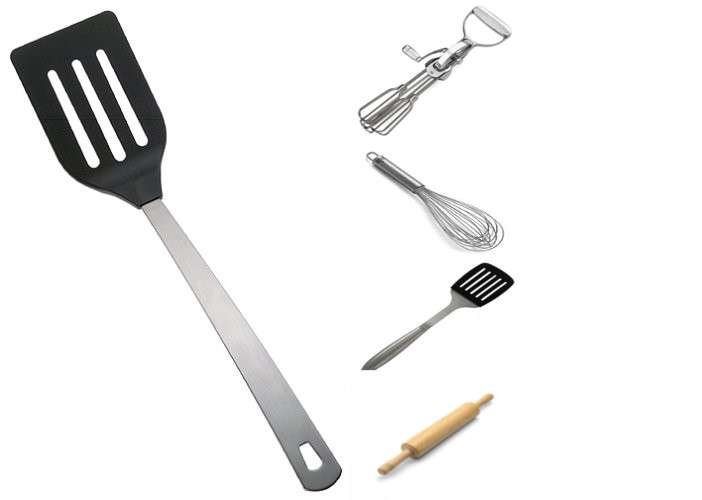（从来没有进过厨房？现在你有机会来测试一下你的单样本学习能力了！右边图像中，哪个是与左边大图片相同类别的？）

• 介绍并定义单样本学习问题
• 描述单样本分类问题的基准，并给出一个其性能的baseline
• 给出一个少样本学习的例子并部分的实现这篇论文中提到的模型
• 指出一些大家通常不会想到的小点子

## 定义这个问题- N类别单样本学习（One-shot Learning）• 现实生活中可能约束更少，可能一张图片并不见得只有一个正确的类别。
• 这个问题很容易泛化到k-shot 学习，我们只需要把每个类别y_{i}yi的单个样本换成k个样本就可以了。
• 当N很的时候，可能有更多可能的类别，所以正确预测类别更难。
• 随机猜的正确率是%.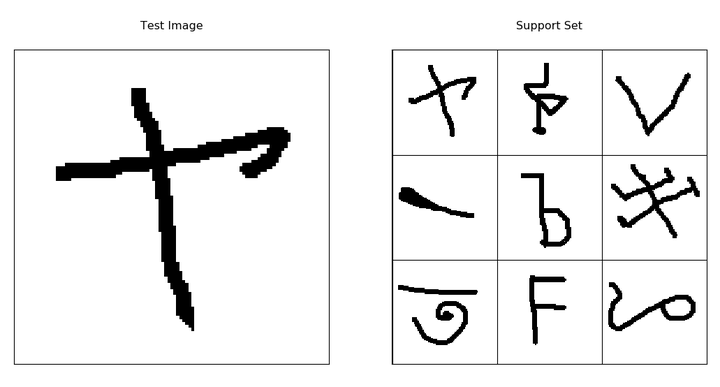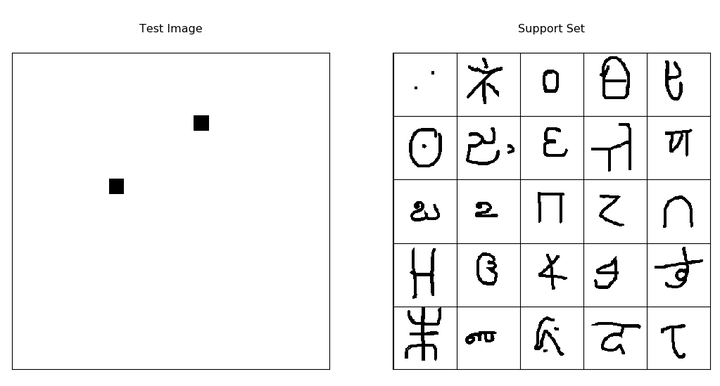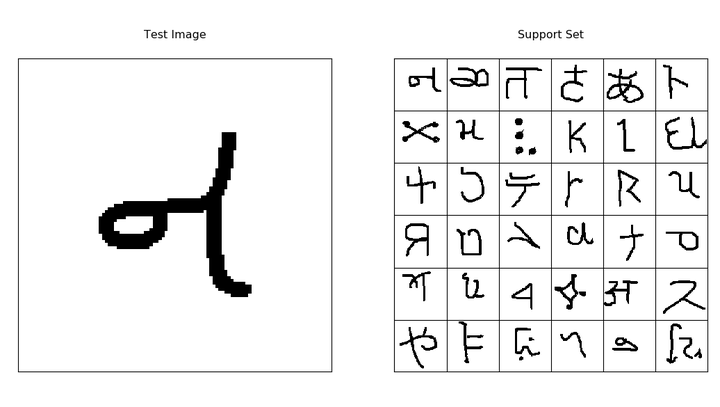## 关于数据集Omniglot！

Omniglot数据集拥有50种，1623类手写字符。对于每类字符仅有20个样本，每个样本分别由不同的人绘制而成，分辨率为105*105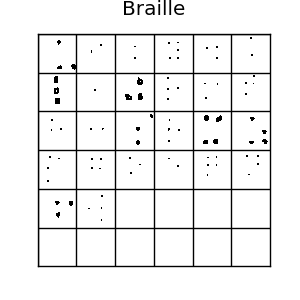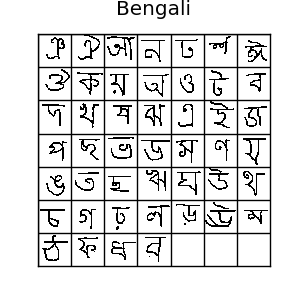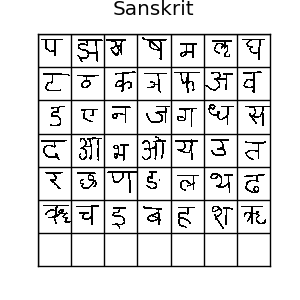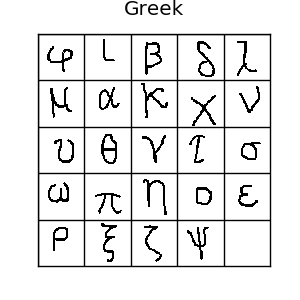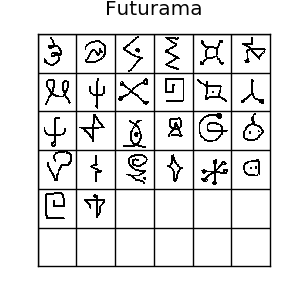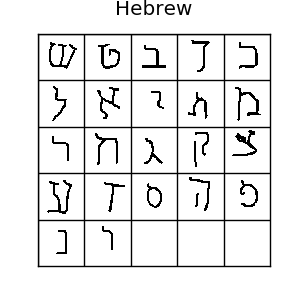## 一个单样本学习的baseline--1近邻Lake等人的Hierarchical Bayesian Program Learning，层次贝叶斯程序学习（以下简称HBPL）得到了大约95.2%的精度，非常不错。我只看懂了30%，但它非常有趣，它与深度学习直接从原始像素上训练相比，是风马牛不相及的，因为：

• HBPL使用笔画数据，而不是仅仅用原始像素
• HBPL在omniglot数据集上学习一个笔画的生成模型，这个算法需要更加复杂的标注，所以不像深度学习能直接从狗、卡车、大脑扫描图以及小铲子等图片的原始像素上去做单样本学习，这种图片也不是由笔画构成的。 Lake等人也指出，人类可以在omniglot数据集20类样本上达到95.5%的精度，仅仅比HBPL高一点。在钻牛角尖思想的引导下，我亲自试验了一下20类任务，达到了97.2%的精度。我并不是做的真正的单样本学习，因为很多符号我本来就认识，因为我熟悉希腊字母、平假名和片假名，我把这些我本来就认识的移除，我还是得到了96.7%的精度。我认为是我从自己吓人的字迹中练就了超人般的字符识别能力。

## 使用深度神经网络来做单样本学习？！

(注意：这里的特征指的是“被用来训练的数据的映射数据“（译者注：例如经过CNN提取到的特征）)

## 孪生网络(Siamese networks)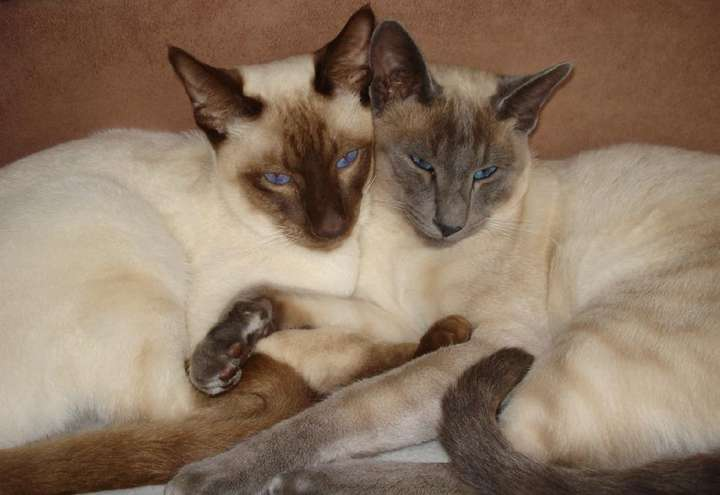## 网络架构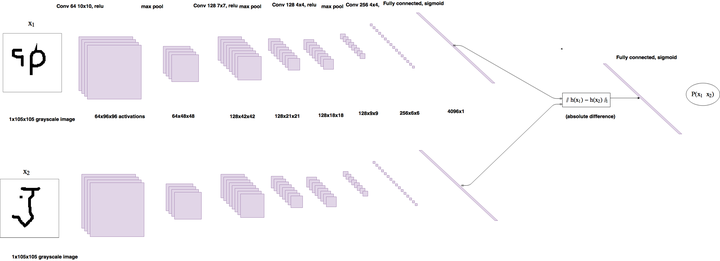## 观察一下：逐对训练的有效的数据集大小对于omniglot中的964类（每类20个样本），这会有185,849,560个可能的配对，这是巨大的！然而，孪生网络需要相同类的和不同类的配对都有。每类E个训练样本，所以每个类别有对，这意味着这里有个相同类别的配对。--对于Omniglot有183,160对。及时183,160对已经很大了，但他只是所有可能配对的千分之一，因为相同类别的配对数量随着E平方级的增大，但是随着C是线性增加。这个问题非常重要，因为孪生网络训练的时候，同类别和不同类别的比例应该是1:1.--或许它表明逐对训练在那种每个类别有更多样本的数据集上更容易训练。

## 代码

from keras.layers import Input, Conv2D, Lambda, merge, Dense, Flatten,MaxPooling2D
from keras.models import Model, Sequential
from keras.regularizers import l2
from keras import backend as K
from keras.losses import binary_crossentropy
import numpy.random as rng
import numpy as np
import os
import dill as pickle
import matplotlib.pyplot as plt
from sklearn.utils import shuffle

def W_init(shape,name=None):
"""Initialize weights as in paper"""
values = rng.normal(loc=0,scale=1e-2,size=shape)
return K.variable(values,name=name)
#//TODO: figure out how to initialize layer biases in keras.
def b_init(shape,name=None):
"""Initialize bias as in paper"""
values=rng.normal(loc=0.5,scale=1e-2,size=shape)
return K.variable(values,name=name)

input_shape = (105, 105, 1)
left_input = Input(input_shape)
right_input = Input(input_shape)
#build convnet to use in each siamese 'leg'
convnet = Sequential()
kernel_initializer=W_init,kernel_regularizer=l2(2e-4)))
kernel_regularizer=l2(2e-4),kernel_initializer=W_init,bias_initializer=b_init))
#encode each of the two inputs into a vector with the convnet
encoded_l = convnet(left_input)
encoded_r = convnet(right_input)
#merge two encoded inputs with the l1 distance between them
L1_distance = lambda x: K.abs(x-x)
both = merge([encoded_l,encoded_r], mode = L1_distance, output_shape=lambda x: x)
prediction = Dense(1,activation='sigmoid',bias_initializer=b_init)(both)
siamese_net = Model(input=[left_input,right_input],output=prediction)
#optimizer = SGD(0.0004,momentum=0.6,nesterov=True,decay=0.0003)

#//TODO: get layerwise learning rates and momentum annealing scheme described in paperworking
siamese_net.compile(loss="binary_crossentropy",optimizer=optimizer)

siamese_net.count_params()


class Siamese_Loader:
def __init__(self,Xtrain,Xval):
self.Xval = Xval
self.Xtrain = Xtrain
self.n_classes,self.n_examples,self.w,self.h = Xtrain.shape
self.n_val,self.n_ex_val,_,_ = Xval.shape

def get_batch(self,n):
"""Create batch of n pairs, half same class, half different class"""
categories = rng.choice(self.n_classes,size=(n,),replace=False)
pairs=[np.zeros((n, self.h, self.w,1)) for i in range(2)]
targets=np.zeros((n,))
targets[n//2:] = 1
for i in range(n):
category = categories[i]
idx_1 = rng.randint(0,self.n_examples)
pairs[i,:,:,:] = self.Xtrain[category,idx_1].reshape(self.w,self.h,1)
idx_2 = rng.randint(0,self.n_examples)
#pick images of same class for 1st half, different for 2nd
category_2 = category if i >= n//2 else (category + rng.randint(1,self.n_classes)) % self.n_classes
pairs[i,:,:,:] = self.Xtrain[category_2,idx_2].reshape(self.w,self.h,1)
return pairs, targets

"""Create pairs of test image, support set for testing N way one-shot learning. """
categories = rng.choice(self.n_val,size=(N,),replace=False)
indices = rng.randint(0,self.n_ex_val,size=(N,))
true_category = categories
ex1, ex2 = rng.choice(self.n_examples,replace=False,size=(2,))
test_image = np.asarray([self.Xval[true_category,ex1,:,:]]*N).reshape(N,self.w,self.h,1)
support_set = self.Xval[categories,indices,:,:]
support_set[0,:,:] = self.Xval[true_category,ex2]
support_set = support_set.reshape(N,self.w,self.h,1)
pairs = [test_image,support_set]
targets = np.zeros((N,))
targets = 1
return pairs, targets

def test_oneshot(self,model,N,k,verbose=0):
"""Test average N way oneshot learning accuracy of a siamese neural net over k one-shot tasks"""
pass
n_correct = 0
if verbose:
print("Evaluating model on {} unique {} way one-shot learning tasks ...".format(k,N))
for i in range(k):
probs = model.predict(inputs)
if np.argmax(probs) == 0:
n_correct+=1
percent_correct = (100.0*n_correct / k)
if verbose:
print("Got an average of {}% {} way one-shot learning accuracy".format(percent_correct,N))
return percent_correct


evaluate_every = 7000
loss_every=300
batch_size = 32
N_way = 20
n_val = 550
best = 76.0
for i in range(900000):
loss=siamese_net.train_on_batch(inputs,targets)
if i % evaluate_every == 0:
if val_acc >= best:
print("saving")
siamese_net.save('PATH')
best=val_acc

if i % loss_every == 0:
print("iteration {}, training loss: {:.2f},".format(i,loss))


## 结果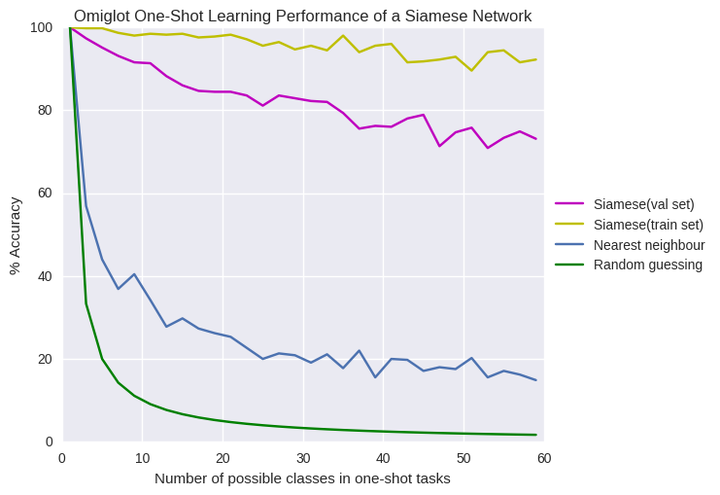## 讨论

Matching Networks for One Shot learning这篇论文就是做这个的。它们使用深度模型来端到端的学习一个完整的近邻分类器，而不是学习相似度函数，直接在单样本任务上训练，而不是在一个图像对上。 Andrej Karpathy’s notes很好的解释了这个问题。因为你正在学习机器分类，所以你可以把他视为元学习（meta learning）。One-shot Learning with Memory-Augmented Neural Networks 这篇论文解释了单样本学习与元学习的关系，它在omniglot数据集上训练了一个记忆增强网络，然而，我承认我看不懂这篇论文。

## 下一步是啥？

omniglot数据集是2015年的了，现在已经有了可拓展机器学习算法在特定的单样本学习上达到了人类的水平。希望未来有一天omniglot数据集会像mnist之于监督学习那样，成为单样本学习的标准检测数据集。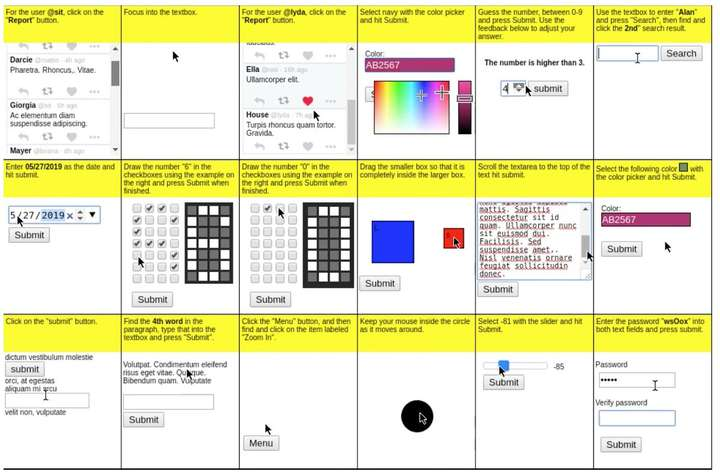OpenAI的比特世界

1. 建立一个神经网络，它可以映射当前状态和一个序列状态（人类示范）到一个动作
2. 把这个模型在人类示范动作和微小的变动任务对上训练，目标是让模型可以基于第一个示范来复现第二个示范动作。

06-08293
09-1336304-032万+
05-13510
04-211480
04-011万+
01-035277
06-14159